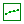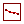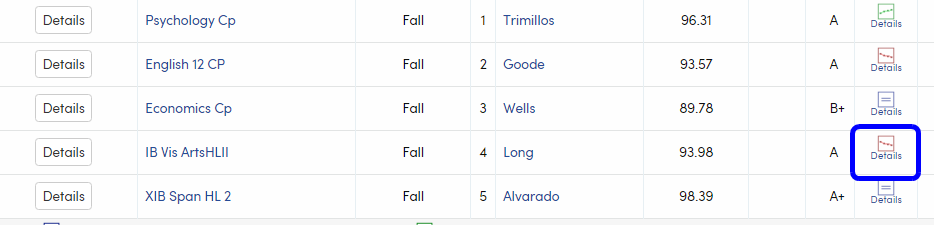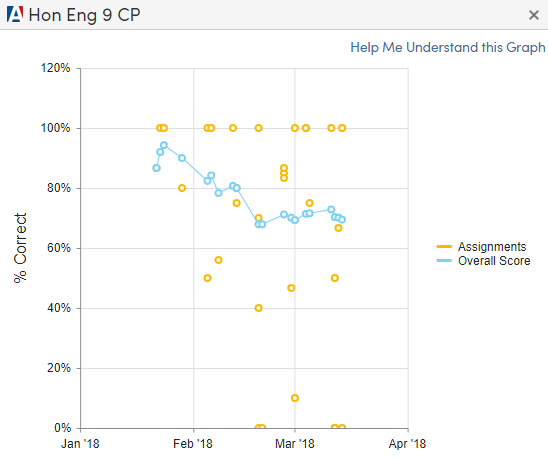## The Overall Score Trend

The Gradebook Trend icon is a quick visual indicator that predicts the student's future overall gradebook score based on existing scores. Details about the calculations used to make the prediction are provided later in this article. The Trend will display only for non-rubric gradebooks and only if the student has at least 10 assignment scores in that gradebook. The icon will display one of three possible trends:

• If the student's overall score is trending up, the icon will show a green, upward slope• If the student's overall score is trending down, the icon will show a red, downward scope• If the student's overall score is trending the same (within 1%), the icon will show a blue equals signIf you hover over the icon, text will display to inform you of the predicted future overall score.This trend is especially valuable to students, parents, and educators because it can be used as a tool to help the student see how their performance is affected by the scores of their assignments. To see the detail, simply click on the icon and you will be presented with a graph showing both the overall trend and each individual assignment score. The overall trend serves as an indicator of the overall performance in the gradebook and how assignments over time affect the overall grade. It is very easy to see how a single missed assignment can greatly impact the overall score and trend of a student's performance.

NOTE: The Gradebook Trend is only for non-rubric gradebooks. For information on how standards-based rubric grades are calculated please see Rubric Standards-Based Gradebook Calculation Explanation.

## The Detailed Graph

When you click the icon, you can see the full graph:Each gold point plotted on the graph represents a specific assignment score. Each blue point on the graph represents the overall score on that date as an average of all assignments up to that point.

If you hover over an individual assignment or overall score, a popup will give you more information for that specific item.

Assignment Detail on hoverOverall Score Detail on hover## Explanation of Calculations

The trend uses a linear regression algorithm to predict what the next overall score for the student will be based upon the historical performance of the student. To be exact, we use either the past 10 overall scores, or the past 25% of overall scores, whichever has the greater number of scores to analyze. Linear regression is a fancy name for prediction. It takes in a range of numbers that are ordered in a specific sequence, then predicts what the next score is going to be and returns the variance or difference between what the range of scores should be and what the predicted next score is. This difference tells you how far away the next number is from the slope or trend of the previous scores.

Let's look at an example. Nick is taking Algebra and has the following scores on his assignments:

 Assign # 1 2 3 4 5 6 7 8 9 10 11 12 Possible 35 10 5 100 10 27 10 5 40 10 40 20 Earned 26 10 5 67 8 20 7 0 14 8 32 15 Score % 74.3 100 100 67 80 74.1 70 0 35 80 80 75 Overall % 74.3 80 82 72 72.5 72.7 72.6 70.8 64.9 65.5 67.5 67.9

His overall scores will be calculated by adding up all the points earned, dividing that by adding up all the points possible, and multiplying that by 100. Therefore, the first of the overall scores will be 74.3% because 26 ÷ 35 is 74.3%. The second overall score will be 80% since (26 + 10) ÷ (35 + 10) = 80%. The third overall score will be (26 + 10 + 5) ÷ (35 + 10 +5) = 82%, and so on.

Using our algorithm (linear regression), we can predict that the next value will be 63.5%. We compare that number against the average of the last four overall scores (64.9 + 65.5 + 67.5 + 67.9) ÷ 4 = 66.4 and we can see that 63.5 is less than 66.4, so this grade is trending down. The trend is down even though we've seen some leveling out lately. The scores at the beginning really set the stage for the trend. This could all change depending on the next couple of assignments, though. When your score is high, one missed assignment will cause your grade to trend down. That is why it is possible for a student to have an overall mark of A in the class and still be trending down.

To allow for some wiggle room, we have the trend difference threshold set to 1%. For example, if the predicted trend is 91.5% and the average of the past four overall scores is 90.6% (0.9% difference) then the trend icon will show an equals sign rather than trending up.

Hopefully, this has been helpful in understanding how we calculate the trend. We hope that this can be used as one measurement to help keep students on track for success.